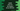# Kotlin example program to find out the largest element in an array# Kotlin example program to find out the largest element in an array :

In this Kotlin example program, we will learn how to find out the largest element in an array of numbers. This is a simple example program that will show you how to find out the largest number in an array. Let’s take a look at the program :

## Kotlin Program :

``````fun main(args: Array) {
//1
val numberArray: IntArray = intArrayOf(10, 20, 30, 40, 50)

//2
var largestElement = numberArray

//3
for (n in numberArray) {
//4
if (largestElement < n)
largestElement = n
}

//5
println("The largest element in the array is %d".format(largestElement))
}``````

### Output :

``The largest element in the array is 50``

### Explanation :

_The commented numbers in the above program denote the step number below : _

1. Create one array of integers with few integer numbers.
2. Create one variable and assign the first element of the array to this number. We will compare this number to all other numbers of the array and find out the largest one.
3. Run one for loop and run each element of the array one by one.
4. Check if the current element is greater than the current largest element or not. If yes, set this value as the largest element.
5. After the loop is completed, print the largest element of the array.

You might also like :